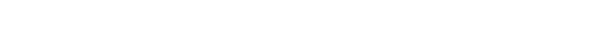# Maximum Likelihood and Maximum Likelihood Estimation

Probability > Maximum Likelihood

## What is Maximum Likelihood?

Maximum Likelihood is a way to find the most likely function to explain a set of observed data.The Poisson family of distributions.In elementary statistics, you are usually given a model to find probabilities. For example, you might be asked to find the probability that X is greater than 2, given the following Poisson distribution:
X ~ Poisson (2.4)
In this example, you are given the parameter, λ, of 2.4 for the Possion distribution. In real life, you don’t have the luxury of having a model given to you: you’ll have to fit your data to a model. That’s where Maximum Likelihood (MLE) comes in.

MLE takes known probability distributions (like the normal distribution) and compares data sets to those distributions in order to find a suitable match for the data. A Family of distributions can have an infinite amount of possible parameters. For example, the mean of the normal distribution could be equal to zero, or it could be equal to ten billion and beyond. Maximum Likelihood Estimation is one way to find the parameters of the population that is most likely to have generated the sample being tested. How well the data matches the model is known as “Goodness of Fit.”

For example, a researcher might be interested in finding out the mean weight gain of rats eating a particular diet. The researcher is unable to weigh every rat in the population so instead takes a sample. Weight gains of rats tend to follow a normal distribution; Maximum Likelihood Estimation can be used to find the mean and variance of the weight gain in the general population based on this sample.

MLE chooses the model parameters based on the values that maximize the Likelihood Function.

## The Likelihood Function

The likelihood of a sample is the probability of getting that sample, given a specified probability distribution model. The likelihood function is a way to express that probability: the parameters that maximize the probability of getting that sample are the Maximum Likelihood Estimators.

Let’s suppose you had a set of random variables X1, X2…Xn taken from an unknown population distribution with parameter Θ. This distribution has a probability density function (PDF) of f(Xi,Θ) where f is the model, Xi is the set of random variables and Θ is the unknown parameter. For the maximum likelihood function you want to know what the most likely value for Θ is, given the set of random variables Xi. The joint probability density function for this example is:Actually finding the maximum likelihood function involves calculus. More specifically, maximizing the PDF. If you aren’t familiar with maximizing functions, you might like this Wolfram Calculator.

Next: EM Algorithm.

CITE THIS AS:
Stephanie Glen. "Maximum Likelihood and Maximum Likelihood Estimation" From StatisticsHowTo.com: Elementary Statistics for the rest of us! https://www.statisticshowto.com/maximum-likelihood-estimation/
---------------------------------------------------------------------------Need help with a homework or test question? With Chegg Study, you can get step-by-step solutions to your questions from an expert in the field. Your first 30 minutes with a Chegg tutor is free!